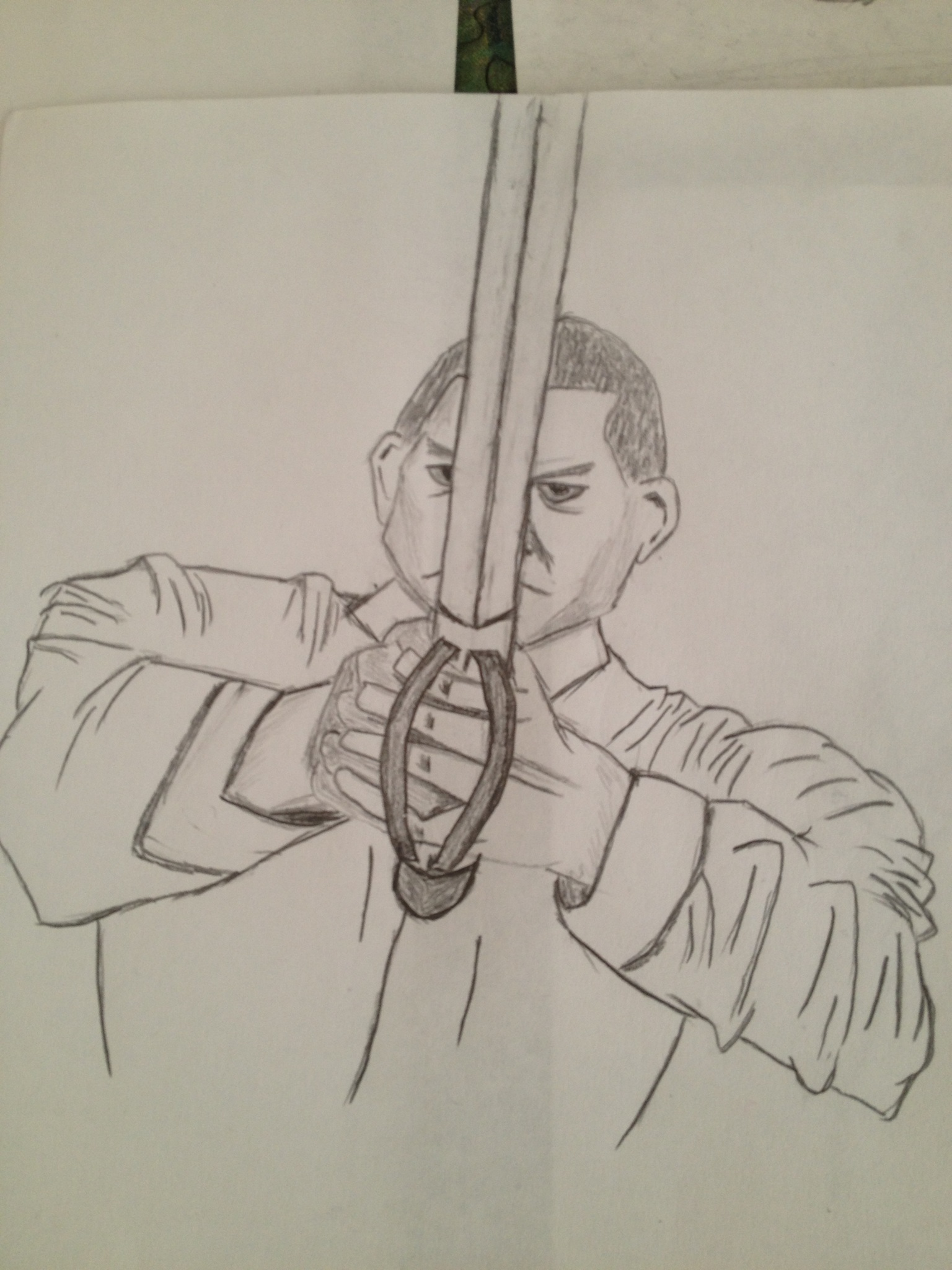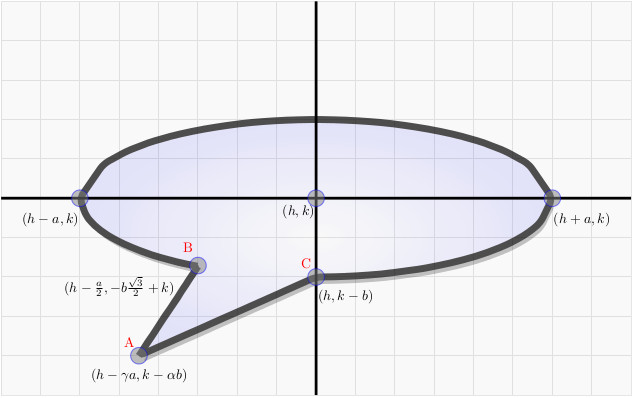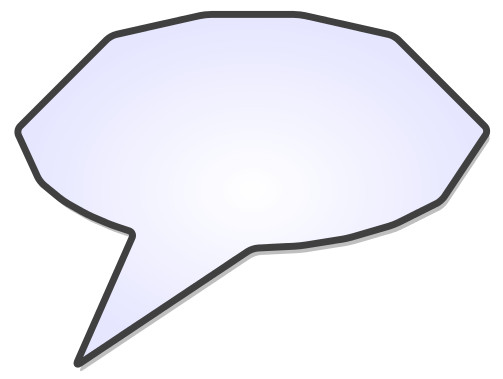# Cartoon Voice Bubbles

One of the things I want to do at some point is make a web comic. I’ve taken up drawing this year. If you want to see the progress, you can follow the artwork here.

Here is one of my favorites:But to make a comic, I need to be able to have artwork that renders nicely on the screen. Additionally, I also have to have consistency in design. Recurring characters need to maintain their appearance over panels and over strips. Some general story-telling is also required.

Of course, my characters are going to have to speak at point. They’ll possibly speak math-y stuff or perhaps quip the things I say in #rantchat. A standard way of showing characters speaking is to use voice bubbles. I’m still hunting around for design. But for something like voice bubbles and thought bubbles, I want a general template. And given that I can program, know a thing or two about math, I figured, what the hey, let’s put those skills to work towards that end.

A classical voice bubble can be viewed as an ellipse with a “fin” protruding in the direction of the character / object that is speaking. An ellipse, generally, is given as $$\frac{(x – h)^{2}}{a^{2}} + \frac{(y-k)^{2}}{b^{2}} = 1$$ where $$(h,k)$$ is the center, $$a$$ and $$b$$ are scale parameters that define the ellipse’s shape. When $$a = b$$ we have a circle.

So, if I want to make a voice bubble programmatically, I have to draw an ellipse and also draw the protrusion. So, to do this, it helps to make a sketch and label the points we’re interested in.You will notice that there are three special points labeled, $$A, B, C$$ with coordinates given relative to the center $$(h,k)$$. You will also notice that this ellipse is drawn “short and fat”. Short-and-fat ellipses have $$a > b$$ and that’s a standard way to draw a voice bubble.

First let’s take a look at point $$B$$ with coordinate $$(h – \frac{a}{2}, -b\frac{\sqrt{3}}{2} + k)$$

$$B$$ is a design choice. Where do I want to break my ellipse? I figured a good break point would be halfway between the center and the far left endpoint in the horizontal direction. This gives the $$x$$-coordinate of $$h – \frac{a}{2}$$. Once we know $$x$$ then it’s just a matter of solving for $$y$$ from the equation of the ellipse, remembering to take the negative $$y$$-coordinate. So this fixes $$B$$.

Next, I made a design choice for $$C$$. I just made $$C$$ the lowest point on the ellipse. That happens at $$(h,k-b)$$.

Finally, and most importantly we have the point $$A$$! This is the third point in the not-closed-off “triangle” $$BAC$$. The question is where should $$A$$ be? Notice that the coordinate given for $$A$$ is $$(h – \gamma a, k – \alpha b)$$ The parameters $$\gamma$$ and $$\alpha$$ control where $$A$$ will be. I have imposed a constraint that $$\alpha > 1$$ since I want the fin to be below $$C$$. Varying $$\alpha$$ lets me control where the fin will be vertically. Varying $$\gamma$$ lets me control where the fin will be horizontally. Technically, there is a constraint on $$\gamma$$ as well in that I don’t it to be so extreme that the fin will so far to the right that it will cross over the voice bubble. But for my purposes, I have a second voice bubble that points to the right. So if I wanted a right point bubble, I would just reflect the left bubble over the vertical axis.

Now what we have to do is figure out some equations. We need three equations: an equation for the line connecting $$A$$ and $$B$$, an equation for the line connecting $$A$$ and $$C$$, and an equation for the ellipse so that it traverses from $$B$$ to $$C$$.

If I use $$A_{x}, A_{y}$$ to represent the $$x$$ and $$y$$ coordinates of point $$A$$, respectively and similarly so for $$B$$ and $$C$$, then the segment $$AB$$ for $$A_{x} \leq x \leq B_{x}$$ is given as $$y_{AB} = m_{AB}(x – A_{x}) + A_{y}$$ where $$m_{AB} = \frac{B_{y} – A_{y}}{B_{x} – A_{x}}$$

Similarly, $$y_{AC} = m_{AC}(x – A_{x}) + A_{y}$$ with $$A_{x} \leq x \leq C_{x}$$

So that takes care of the fin. And the ellipse we already know to be $$\frac{(x – h)^{2}}{a^{2}} + \frac{(y-k)^{2}}{b^{2}} = 1$$ where we recognize that for $$B_{x} \leq x \leq C_{x}$$ we should only take the positive portion.

Now that we have the formulas down, we just have to program it. I used Python because it was straightforward and had the output write to a $$\LaTeX$$ file. Then combined with a little bit of tikz magic, I get voice bubbles! The eagle-eyed observer will notice that there is some “jaggedness” to the ellipse. This is purposeful because I didn’t want to have too smooth of a bubble. I can make it smoother by increasing the number of sampling points since the ellipse is constructed by connecting lines at the sampling points.

All the programming and output magic for $$\LaTeX$$ import is done in a function I called leftbubble(h,k,a,b,alpha,n). Here n is (loosely) the number of sampling points I want. Partially out of laziness and partially because the look I got was what I wanted, $$n$$ is the number of sampling points per section of the voice bubble I want to sample. And because I am being mindful of the order in which I have to traverse the ellipse, I sample from $$B$$ to $$(h-a,k)$$ and then to $$(h+a,k)$$ and then to $$C$$. So, the upper half of the ellipse will be more jagged.

Here are a few samples of left voice bubbles with $$\gamma = \frac{3}{4}$$ and varying $$a, b,\alpha$$ and $$n$$. The figure caption gives the parameters so you can see what happens as the inputs are changed.Voice Bubble 3: leftbubble(0,0,6,3,2,5) (notice the jagged effect of reducing the “sampling” points from 20 to 5)

There are other things that we can do here. If I didn’t want the fin to be triangular, I could change it’s characteristic so that it would be parabolic. I can also rotate the voice bubble if I needed to. It would just be a matter of adding a few extra lines of code to handle the transformation.

How clean and crisp of a design I want to make is up to me, but now at least I have a general, automatic way of making thematically consistent elements of a comic. I also have something similar for “thought bubbles”, but I’ll write about that later. Perhaps even demo it with a comic!